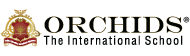# Compound Interest Calculator

What Is Compound Interest?
Compound interest is the interest earned on your deposit, along with the cumulative interest over a period of time. The word ‘compounding’ means interest is added to the present principal amount.
Example:
For instance, Rohan has taken a Rs. 2000 loan from the bank at the compound interest rate of 15% per year for two years. After the first year, the amount Rohan owes to the bank would be:
Interest calculated in Year 1: 2000 15% 1 year=300 So the interest Rohan owes the bank by the end of the year is rupees, 2300 By the second year, the interest is calculated on the total amount, i.e., the principal amount and the additional interest, i.e., 2000 plus 300. In other words, the interest of 15% is applied to the total amount due at the end of the first year.
Interest Calculated in Year 2: (2000+300)15% 1 year =345 Hence, the interest Rohan owes the bank by the end of the second year will be rupees 345. And the total amount he owes the banks would be 2645.
How Is The Compound Interest Calculated?
The amount can be compounded either monthly, quarterly, half-yearly, yearly, or even daily or weekly.
The formula to calculate compound interest is:
A= P (1+r/n)nt
Where:
• A = amount
• P = principal
• r = rate of interest
• n = the number of times interest has increased in a given year
• t = time (in years)
Example
Mr. Aditya invested Rs. 60,000 with an interest rate of 12% for a term of five years. Calculate the compound interest.
Answer:60,000 x 12/100 = Rs. 7,200
Similarly, the interest for Aditya’s second year will be calculated on the amount, i.e., 60,000 + 7200 = 67,200
What Is A Compound Interest Calculator?
A compound interest calculator is a web-based tool that assists you in calculating compound interest.
The compound interest calculator online gives accurate results, for which you need to follow the steps given below:
Step 1: Type the values, like the principal amount, interest rate, and the term of the loan or the time in which the amount has to be repaid.
Step 2: Click the ‘calculate’ button to get the compound interest and the total amount to be paid back.
This compound interest calculator online can help you accurately calculate it. Calculate the power required to do a work of 2000 J in 45 seconds of time.
How To Calculate Compound Interest Monthly?
To calculate compound interest per month, the monthly compound interest formula is used. The interest is added to the principal amount each month. Total compound interest is the final amount that excludes the principal amount.
CI=P{1 + r12}12t-P
The principal amount is compounded 12 times a year since there are 12 months in a year.
How To Calculate Compound Interest Quarterly?
When the amount is compounded quarterly(n = 4), the formula is:
A=P(1+r4)4t

## FAQs

##### How can you easily calculate compound interest?

| K12 Techno Services ®

ORCHIDS - The International School | Terms | Privacy Policy | Cancellation